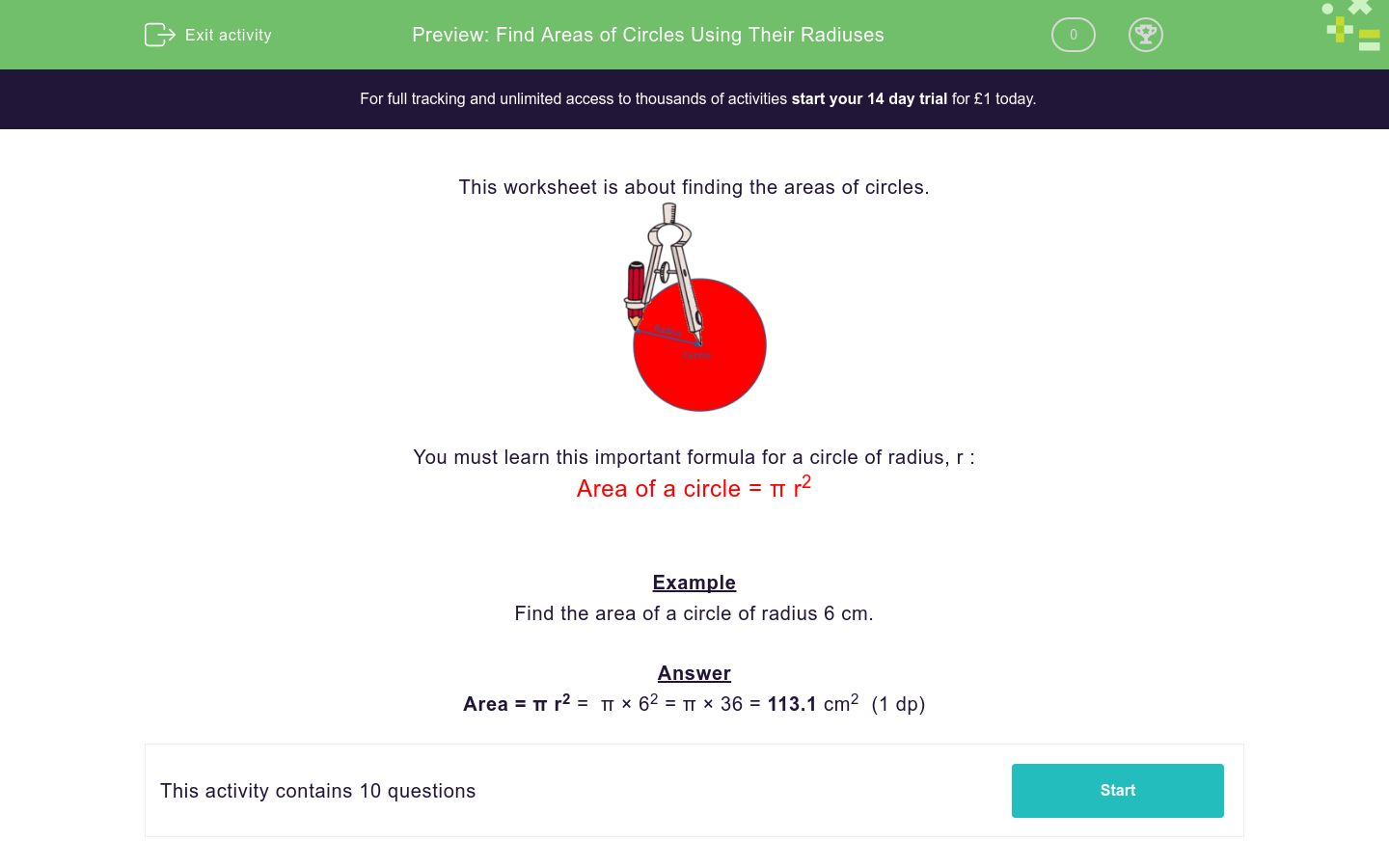# Find Areas of Circles Using Their Radiuses

In this worksheet, students find the areas of circles given their radiuses.Key stage:  KS 3

Curriculum topic:  Geometry and Measures

Curriculum subtopic:  Solve Problems Involving Perimeters and Areas of 2D Shapes

Difficulty level:### QUESTION 1 of 10

This worksheet is about finding the areas of circles.You must learn this important formula for a circle of radius, r :

Area of a circle = π r2

Example

Find the area of a circle of radius 6 cm.

Answer

Area = π r2 =  π × 62 = π × 36 = 113.1 cm2  (1 dp)

Find the area of a circle of radius 10 cm in cm2.

Just write the number to 1 decimal place.

Find the area of a circle of radius 2 cm in cm2.

Just write the number to 1 decimal place.

Find the area of a circle of radius 5 cm in cm2.

Just write the number to 1 decimal place.

Find the area of a circle of radius 6 cm in cm2.

Just write the number to 1 decimal place.

Find the area of a circle of radius 16 cm in cm2.

Just write the number to 1 decimal place.

Find the area of a circle of radius 25 cm in cm2.

Just write the number to 1 decimal place.

Find the area of a circle of radius 5.8 cm in cm2.

Just write the number to 1 decimal place.

Find the area of a circle of radius 12.4 cm in cm2.

Just write the number to 1 decimal place.

Find the area of a circle of radius 0.5 cm in cm2.

Just write the number to 1 decimal place.

Find the area of a circle of radius 3.99 cm in cm2.

Just write the number to 1 decimal place.

• Question 1

Find the area of a circle of radius 10 cm in cm2.

Just write the number to 1 decimal place.

CORRECT ANSWER
314.2
EDDIE SAYS

Area = π x r2

Area = π x 102

• Question 2

Find the area of a circle of radius 2 cm in cm2.

Just write the number to 1 decimal place.

CORRECT ANSWER
12.6
EDDIE SAYS

Area = π x r2

Area = π x 22

• Question 3

Find the area of a circle of radius 5 cm in cm2.

Just write the number to 1 decimal place.

CORRECT ANSWER
78.5
EDDIE SAYS

Area = π x r2

Area = π x 52

• Question 4

Find the area of a circle of radius 6 cm in cm2.

Just write the number to 1 decimal place.

CORRECT ANSWER
113.1
EDDIE SAYS

Area = π x r2

Area = π x 62

• Question 5

Find the area of a circle of radius 16 cm in cm2.

Just write the number to 1 decimal place.

CORRECT ANSWER
804.2
EDDIE SAYS

Area = π x r2

Area = π x 162

• Question 6

Find the area of a circle of radius 25 cm in cm2.

Just write the number to 1 decimal place.

CORRECT ANSWER
1963.5
EDDIE SAYS

Area = π x r2

Area = π x 252

• Question 7

Find the area of a circle of radius 5.8 cm in cm2.

Just write the number to 1 decimal place.

CORRECT ANSWER
105.7
EDDIE SAYS

Area = π x r2

Area = π x 5.82

• Question 8

Find the area of a circle of radius 12.4 cm in cm2.

Just write the number to 1 decimal place.

CORRECT ANSWER
483.1
EDDIE SAYS

Area = π x r2

Area = π x 12.42

• Question 9

Find the area of a circle of radius 0.5 cm in cm2.

Just write the number to 1 decimal place.

CORRECT ANSWER
0.8
EDDIE SAYS

Area = π x r2

Area = π x 0.52

• Question 10

Find the area of a circle of radius 3.99 cm in cm2.

Just write the number to 1 decimal place.

CORRECT ANSWER
50.0
EDDIE SAYS

Area = π x r2

Area = π x 3.992

---- OR ----

Sign up for a £1 trial so you can track and measure your child's progress on this activity.

### What is EdPlace?

We're your National Curriculum aligned online education content provider helping each child succeed in English, maths and science from year 1 to GCSE. With an EdPlace account you’ll be able to track and measure progress, helping each child achieve their best. We build confidence and attainment by personalising each child’s learning at a level that suits them.

Start your £1 trial